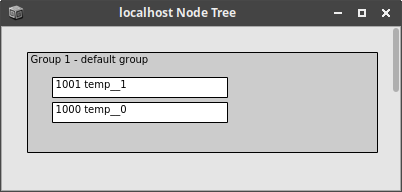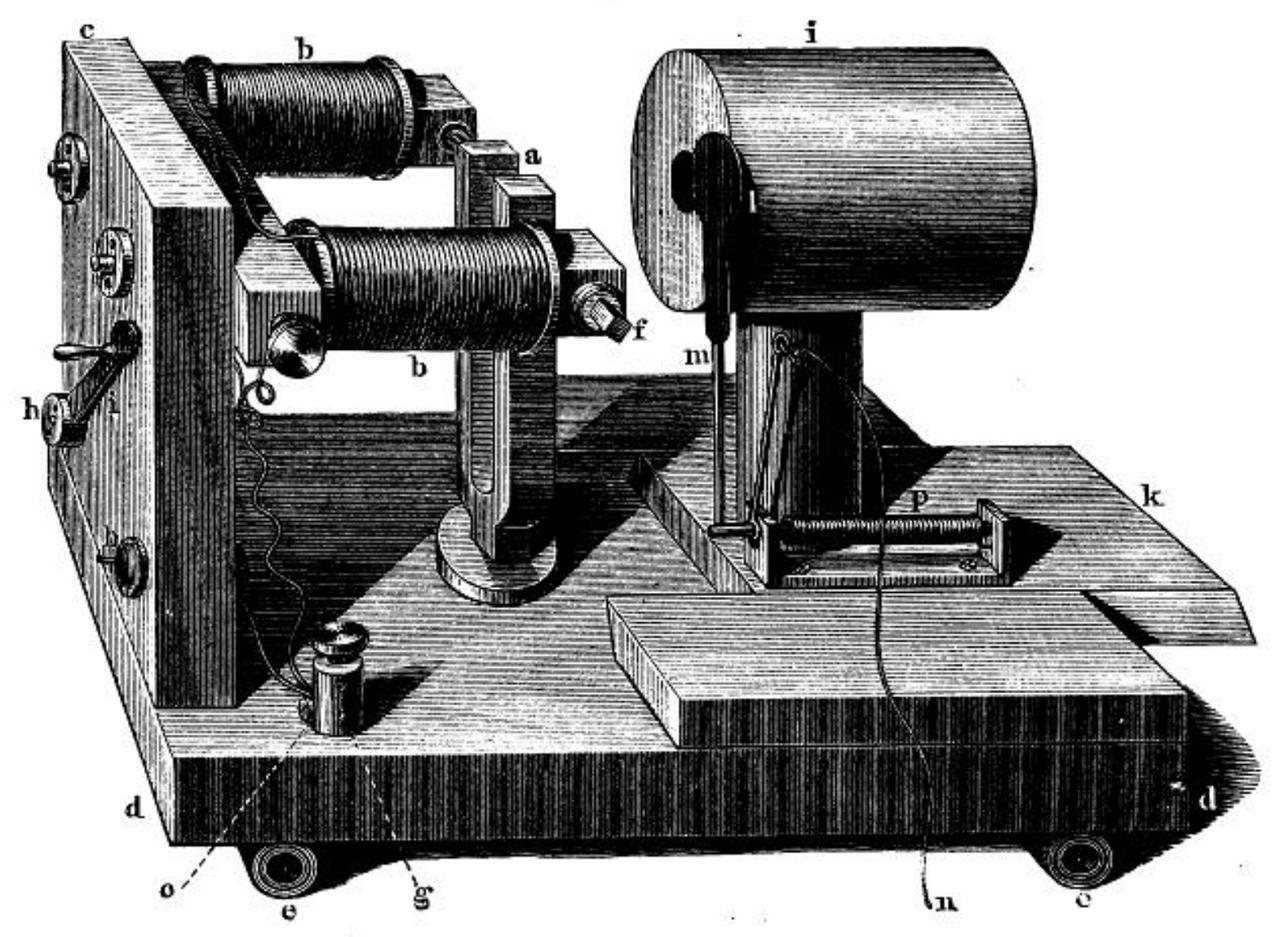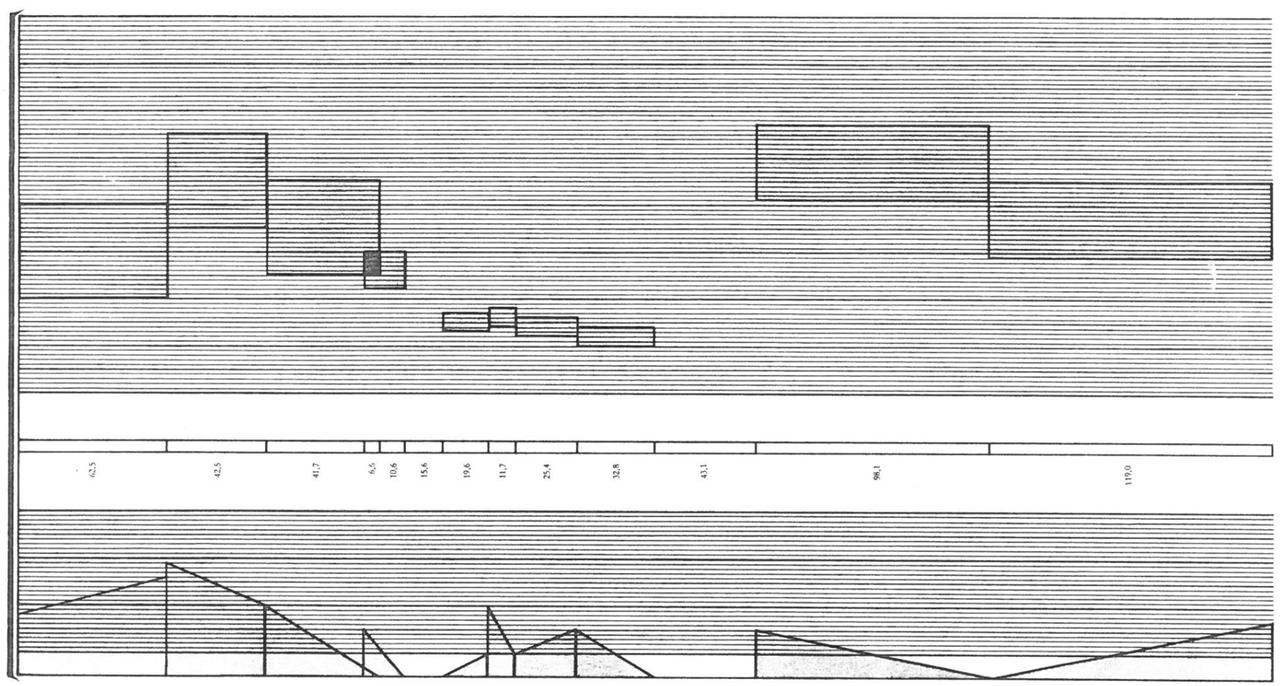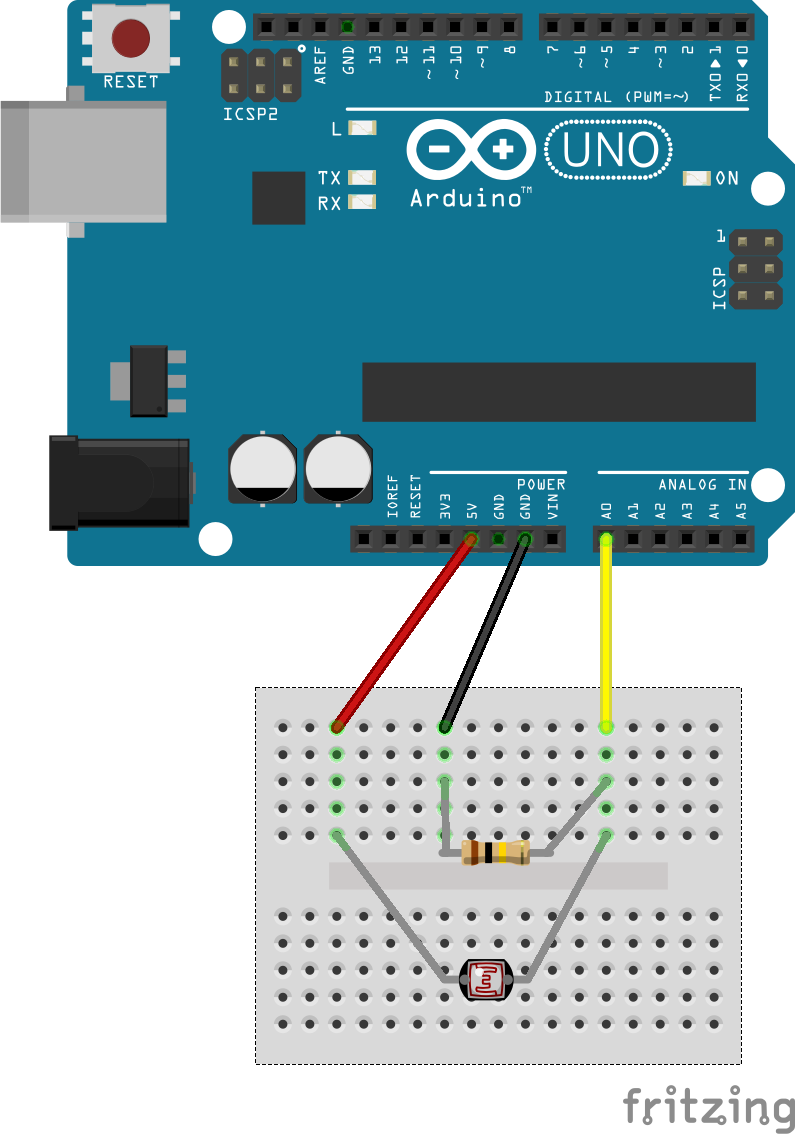# Combining Nodes in SuperCollider

## Creating and Connecting Nodes

Audio buses can be used to connect synth nodes. In this example we will create two nodes - one for generating a sound and one for processing it. First thing is an audio bus:

~aBus = Bus.audio(s,1);


The ~osc node generates a sawtooth signal and the output is routed to the audio bus:

~osc = {arg out=1; Out.ar(out,Saw.ar())}.play;

~osc.set(\out,~aBus.index);


The second node is a simple filter. Its input is set to the index of the audio bus:

~lpf = {arg in=0; Out.ar(0, LPF.ar(In.ar(in),100))}.play;

~lpf.set(\in,~aBus.index);


Warning

Although everything is connected, there is no sound at this point. SuperCollider can only process such chains if the nodes are arranged in the right order. The filter node can be moved after the oscillator node:

## Moving NodesNode Tree before moving the processor node.

The moveAfter() function is a quick way for moving a node directly after a node specified as the argument. The target node can be either referred to by its node index or by the related name in sclang:

~lpf.moveAfter(~osc)Node Tree after moving the processor node.

# More APIs

There are many more APIs which can be used for real time or off line sonification. Several projects and meta sites list examples by category:

## NASA

NASA offers a great variety of open APIs with data from astronomy: https://api.nasa.gov/

# Digital Waveguides: Discrete Wave Equation

## Wave Equation for Ideal Strings

The ideal string results in an oscillation without losses. The differential wave-equation for this process is defined as follows. The velocity $c$ determines the propagation speed of the wave and this the frequency of the oscillation.

\begin{equation*} \frac{\partial^2 y}{\partial t^2} = c^2 \frac{\partial^2 y}{\partial x^2} \end{equation*}

A solution for the different equation without losses is given by d'Alembert (1746). The oscillation is composed of two waves - one left-traveling and one right traveling component.

\begin{equation*} y(x,t) = y^+ (x-ct) + y^- (x+ct)$\end{equation*} • $y^+$ = left traveling wave • $y^-$ = right traveling wave ## Tuning the String The velocity $c$ depends on tension $K$ and mass-density $\epsilon$ of the string: \begin{equation*} c^2 = \sqrt{\frac{K}{\epsilon}} = \sqrt{\frac{K}{\rho S}} \end{equation*} With tension $K$, cross sectional area $S$ and density $\rho$ in ${\frac{g}{cm^3}}$. Frequency $f$ of the vibrating string depends on the velocity and the string length: \begin{equation*} f = \frac{c}{2 L} \end{equation*} ## Make it Discrete For an implementation in digital systems, both time and space have to be discretized. This is the discrete version of the above introduced solution: \begin{equation*} y(m,n) = y^+ (m,n) + y^- (m,n) \end{equation*} For the time, this discretization is bound to the sampling frequency $f_s$. Spatial sample distance $X$ depends on sampling-rate $f_s = \frac{1}{T}$ and velocity $c$. • $t = \ nT$ • $x = \ mX$ • $X = cT$ # Faust: MIDI ## Using MIDI CC Using MIDI in Faust requires only minor additions to the code and compiler arguments. For first steps it can be helpful to control single synth parameters with MIDI controllers. This can be configured via the UI elements. The following example uses MIDI controller number 48 to control the frequency of a sine wave by adding [midi:ctrl 48] to the hslider parameters. // midi-example.dsp // // Control a sine wave frequency with a MIDI controller. // // Henrik von Coler // 2020-05-17 import("stdfaust.lib"); freq = hslider("frequency[midi:ctrl 48]",100,20,1000,0.1) : si.smoo; process = os.osc(freq) <: _,_ ;  CC 48 has been chosen since it is the first slider on the AKAI APC mini. If the controller numbers for other devices are not known, they can be found using the PD patch reverse_midi.pd. ## Compiling with MIDI In order to enable the MIDI functions, the compiler needs to be called with an additional flag -midi: $ faust2xxxx -midi midi_example.dsp

This flag can also be combined with the -osc flag to make synths listen to both MIDI and OSC.

## Note Handling & Polyphony

Typical monophonic and polyphonic synth control can be added to Faust programs by defining and mapping three parameters:

• freq

• gain

• gate

When used like in the following example, they will be linked to the parameters of MIDI note on and note off events with a frequency and a velocity.

// midi_trigger.dsp
//
// Henrik von Coler
// 2020-05-17

import("stdfaust.lib");
freq    = nentry("freq",200,40,2000,0.01) : si.polySmooth(gate,0.999,2);
gain   = nentry("gain",1,0,1,0.01) : si.polySmooth(gate,0.999,2);
gate   = button("gate") : si.smoo;

process = vgroup("synth",os.sawtooth(freq)*gain*gate <: _,_);


$faust2xxxx -midi -nvoices 12 midi_trigger.dsp ## MIDI on Linux Faust programs use Jack MIDI, whereas MIDI controllers usually connect via ALSA MIDI. In order to control the synth with an external controller, a bridge is nedded: $ a2jmidi_bridge

The MIDI controller can now connect to the a2j_bridge input, which is then connected to the synth input.

# Faust: Splitting and Merging Signals

## Splitting a Signal

### To Stereo

The <: operator can be used to split a signal into an arbitrary number of branches. This is frequently used to send a signal to both the left and the right channel of a computer's output device. In the following example, an impulse train with a frequency of $5\ \mathrm{Hz}$ is generated and split into a stereo signal.

##import("stdfaust.lib");

// a source signal
signal = os.imptrain(5);

// split signal to stereo in process function:
process = signal <: _,_;


### To Many

The splitting operator can be used to create more than just two branches. The following example splits the source signal into 8 signals:

##To achieve this, the splitting directive can be extended by the desired number of outputs:

process = signal <: _,_,_,_,_,_,_,_;


## Merging Signals

### Merging to Single

The merging operator :> in Faust is the inversion of the splitting operator. It can combine an arbitrary number of signals to a single output. In the following example, four individual sine waves are merged:

##Input signals are separated by commas and then joined with the merging operator.

import("stdfaust.lib");

// create four sine waves
// with arbitrary frequencies
s1 = 0.2*os.osc(120);
s2 = 0.2*os.osc(340);
s3 = 0.2*os.osc(1560);
s4 = 0.2*os.osc(780);

// merge them to two signals
process = s1,s2,s3,s4 :> _;


### Merging to Multiple

Merging can be used to create multiple individual signals from a number of input signals. The following example generates a stereo signal with individual channels from the four sine waves:

##To achieve this, two output signals need to be assigned after merging:

// merge them to two signals
process = s1,s2,s3,s4 :> _,_;


### Exercise

Exercise

Extend the Merging to Single example to a stereo output with individual left and right channels.

# Subtractive Example

The following example uses a continuous square wave generator with different filters for exploring their effect on a harmonic signal.

#### Controls

Pitch (VCF):

Filter Type:

Lowpass Highpass Bandpass Notch (Band Reject)

Cutoff (VFC):

Q (VCF):

Gain (VCA):

t/s

f/Hz

## Early Mechanical

Early use of the Fourier representation, respectively additive synthesis, for modeling musical sounds has been made by Hermann von Helmholtz. He built mechanical devices, based on tuning forks, resonant tubes and electromagnetic excitation for additive synthesis. Von Helmholtz used these devices for investigating various aspects of harmonic sounds, including spectral distribution and relative phases.Tuning forks with resonant tubes (von Helmholtz, 1870, p.183).

## Early Analog

The history of Elektronische Musik started with additive synthesis. In his composition Studie II, Karlheinz Stockhausen composed timbres by superimposing sinusoidal components. In that era this was realized through single sine wave oscillators, tuned to the desired frequency and recorded on tape.

Studie II is the attempt to fully compose music on a timbral level in a rigid score. Stockhausen therefor generated tables with frequencies and mixed tones for creating source material. Fig.1 shows an excerpt from the timeline, which was used to arrange the material. The timbres are recognizable through their vertical position in the upper system, whereas the lower system represents articulation, respectively fades and amplitudes.Fig.1: From the score of Studie II.

## Early Digital

Max Mathews

As mentioned in the Introduction, Max Mathews used additive synthesis to generate the first digitally synthesized pieces of music in the 1950s. In the early 1960s, Mathews had advanced the method to synthesize dynamic timbres, as in Bycicle Built for Two:

Iannis Xenakis

In his Electroacoustic compositions, Iannis Xenakis made use of the UPIC system for additive synthesis (Di Scipio, 1998), as for example in his Mycenae-Alpha (1977).

### References

#### 1870

• Hermann von Helmholtz. Die Lehre von den Tonempfindungen als physiologische Grundlage für die Theorie der Musik, 3. umgearbeitete Ausgabe. Braunschweig: Vieweg, 1870.
[details] [BibTeX▼]

# Sampling & Aliasing: Square Example

For the following example, a sawtooth with 20 partials is used without band limitation. Since the builtin Web Audio oscillator is band-limited, a simple additive synth is used in this case. At a pitch of about $2000 Hz$, the aliases become audible. For certain fundamental frequencies, all aliases will be located at actual multiples of the fundamental, resulting in a correct synthesis despite aliasing. In most cases, the mirrored partials are inharmonic and distort the signal and for higher fundamental frequencies the pitch is fully dissolved.

Pitch (Hz):

Output Gain:

Time Domain:

Frequency Domain:

## Anti-Aliasing Filters

In analog-to-digital conversion, simple anti-aliasing filters can be used to band-limit the input and discard signal components above the Nyquist frequency. In case of digital synthesis, however, this principle can not be applied. When generating a square wave signal with an infinite number of harmonics, aliasing happens instantaneously and can not be removed, afterwards.

## Band Limited Generators

In order to avoid the aliasing, band-limited signal generators are provided in most audio programming languages and environments.

# SuperCollider: Light Dependent Resistor

This example shows how a single sensor can be streamed via serial data from the Arduino to SuperCollider.

The breadboard circuit is the same as in the first Arduino sensor example:## Arduino Code

For the SC example, serial data is sent in a simple way. The additional scaling is optional, but makes it easier to process the data in SuperCollider.

void setup() {

Serial.begin(9600);
}

void loop() {

// scale to 0..1
float voltage   = sensorValue/1024.0 ;

Serial.println(voltage);

}


## SC Code

On Linux, the Arduino's serial interface can be found in the terminal:

\$ ls -l /dev/ttyACM*


On the SC receiver end, a serial port object is initialized with the matching serial interface:

(
p = SerialPort(
"/dev/ttyACM0",
baudrate: 9600,
crtscts: true);
)


A control rate bus is used to visualize the received data and make it accessible to other nodes:

~sensorBUS = Bus.control(s,1);
~sensorBUS.scope;


The actual receiving and decoding of the data happens inside a routine with an infinite loop. It appends incoming characters, until a return character (13) is received. In this case, the assembled string is converted to a Float and written to the sensor bus:

(
r= Routine({
var byte, str, res;
inf.do{|i|
str = "";
while({byte = p.read; byte !=13 }, {
str= str++byte.asAscii;
});
res= str.asFloat;

~sensorBUS.set(res);
});
};
}).play;
)


## External Resources

The SuperCollider Tutorial by Eli Fieldsteel shows a similar solution for getting Arduino sensors into SuperCollider via USB.

## Exercise

Exercise

Create a synth node with a parameter mapped to the sensor bus.

# Asteroids - NeoWs

## NeoWs

At https://api.nasa.gov/, the NASA offers various APIs. This example uses data from the 'Asteroids - NeoWs' RESTful web service, which contains data of near earth Asteroids.

## JSON Data Structure

The JSON data is arraned as an array, featuring the data on 20 celestial bodies, accessible via index:

links {…}
page  {…}
near_earth_objects
0   {…}
1   {…}
2   {…}
3   {…}
4   {…}
5   {…}
6   {…}
7   {…}
8   {…}
9   {…}
10  {…}
11  {…}
12  {…}
13  {…}
14  {…}
15  {…}
16  {…}
17  {…}
18  {…}
19  {…}


## Harmonic Sonification

### Mapping

All entries of the individual Asteroids can be used as synthesis parameters in a sonification system with Web Audio. This example uses two parameters of the Asteroids within an additive synthesis paradigm:

orbital_period       = sine wave frequency
absolute_magnitude_h = sine wave  amplitude